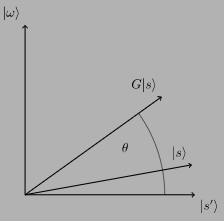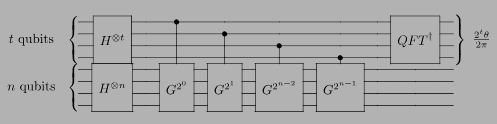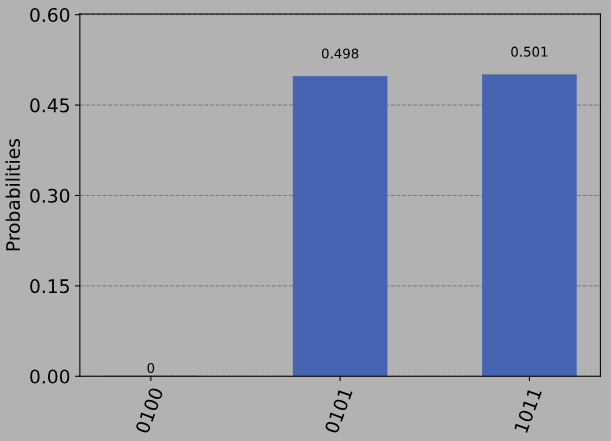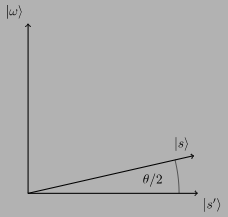# 量子计算

## 1. 概述

### 1.1直觉### 1.2仔细观察

|\omega\rangle |s ' \rangle 基中，我们可以将Grover迭代器写成这样的矩阵:

G = \begin{pmatrix} \cos{\theta} && -\sin{\theta}\\ \sin{\theta} && \cos{\theta} \end{pmatrix}

\begin{pmatrix} -i\\ 1 \end{pmatrix} , \begin{pmatrix} i\\ 1 \end{pmatrix}

|s\rangle = \alpha |\omega\rangle + \beta|s'\rangle## 2. 代码

### 2.1初始化代码

import matplotlib.pyplot as plt
import numpy as np
import math

# importing Qiskit
import qiskit
from qiskit import QuantumCircuit, transpile, assemble, Aer

# import basic plot tools
from qiskit.visualization import plot_histogram


### 2.2 Controlled-Grover迭代

def example_grover_iteration():
"""Small circuit with 5/16 solutions"""
# Do circuit
qc = QuantumCircuit(4)
# Oracle
qc.h([2,3])
qc.ccx(0,1,2)
qc.h(2)
qc.x(2)
qc.ccx(0,2,3)
qc.x(2)
qc.h(3)
qc.x([1,3])
qc.h(2)
qc.mct([0,1,3],2)
qc.x([1,3])
qc.h(2)
# Diffuser
qc.h(range(3))
qc.x(range(3))
qc.z(3)
qc.mct([0,1,2],3)
qc.x(range(3))
qc.h(range(3))
qc.z(3)
return qc


# Create controlled-Grover
grit = example_grover_iteration().to_gate()
grit.label = "Grover"
cgrit = grit.control()


### 2.3逆QFT

def qft(n):
"""Creates an n-qubit QFT circuit"""
circuit = QuantumCircuit(4)
def swap_registers(circuit, n):
for qubit in range(n//2):
circuit.swap(qubit, n-qubit-1)
return circuit
def qft_rotations(circuit, n):
"""Performs qft on the first n qubits in circuit (without swaps)"""
if n == 0:
return circuit
n -= 1
circuit.h(n)
for qubit in range(n):
circuit.cp(np.pi/2**(n-qubit), qubit, n)
qft_rotations(circuit, n)

qft_rotations(circuit, n)
swap_registers(circuit, n)
return circuit


qft_dagger = qft(4).to_gate().inverse()
qft_dagger.label = "QFT†"


### 2.4整合

# Create QuantumCircuit
t = 4   # no. of counting qubits
n = 4   # no. of searching qubits
qc = QuantumCircuit(n+t, t) # Circuit with n+t qubits and t classical bits

# Initialize all qubits to |+>
for qubit in range(t+n):
qc.h(qubit)

# Begin controlled Grover iterations
iterations = 1
for qubit in range(t):
for i in range(iterations):
qc.append(cgrit, [qubit] + [*range(t, n+t)])
iterations *= 2

# Do inverse QFT on counting qubits
qc.append(qft_dagger, range(t))

# Measure counting qubits
qc.measure(range(t), range(t))

# Display the circuit
qc.draw()


## 3.模拟

# Execute and see results
aer_sim = Aer.get_backend('aer_simulator')
transpiled_qc = transpile(qc, aer_sim)
qobj = assemble(transpiled_qc)
job = aer_sim.run(qobj)
hist = job.result().get_counts()
plot_histogram(hist)measured_str = max(hist, key=hist.get)


measured_int = int(measured_str,2)
print("Register Output = %i" % measured_int)


## 4. 求解的个数(M)

\theta = \text{value}\times\frac{2\pi}{2^t}

theta = (measured_int/(2**t))*math.pi*2
print("Theta = %.5f" % theta)


\theta = 4.31969\langle s'|s\rangle = \cos{\tfrac{\theta}{2}}

|s\rangle (计算基态的均匀叠加)可以写成 |\omega\rangle |s'\rangle ，如下:

|s\rangle = \sqrt{\tfrac{M}{N}}|\omega\rangle + \sqrt{\tfrac{N-M}{N}}|s'\rangle

|s\rangle |s'\rangle 的内积为:

\langle s'|s\rangle = \sqrt{\frac{N-M}{N}} = \cos{\tfrac{\theta}{2}}

N\sin^2{\frac{\theta}{2}} = M

N = 2**n
M = N * (math.sin(theta/2)**2)
print("No. of Solutions = %.1f" % (N-M))


m = t - 1 # Upper bound: Will be less than this
err = (math.sqrt(2*M*N) + N/(2**(m+1)))*(2**(-m))
print("Error < %.2f" % err)


def calculate_M(measured_int, t, n):
"""For Processing Output of Quantum Counting"""
# Calculate Theta
theta = (measured_int/(2**t))*math.pi*2
print("Theta = %.5f" % theta)
# Calculate No. of Solutions
N = 2**n
M = N * (math.sin(theta/2)**2)
print("No. of Solutions = %.1f" % (N-M))
# Calculate Upper Error Bound
m = t - 1 #Will be less than this (out of scope)
err = (math.sqrt(2*M*N) + N/(2**(m+1)))*(2**(-m))
print("Error < %.2f" % err)


## 5. 练习

1. 你可以用不同数量的解决方案创建一个oracle吗?量子计数算法的精度如何变化?

2. 你能调整电路以使用更多或更少的计数量子比特来获得不同的结果精度吗?

## 6. 参考文献

 Michael A. Nielsen and Isaac L. Chuang. 2011. Quantum Computation and Quantum Information: 10th Anniversary Edition (10th ed.). Cambridge University Press, New York, NY, USA.

import qiskit.tools.jupyter
%qiskit_version_table


### 版本信息

Qiskit Software Version
Qiskit 0.27.0
Terra 0.17.4
Aer 0.8.2
Ignis 0.6.0
Aqua 0.9.2
IBM Q Provider 0.14.0
System information
Python 3.7.7 (default, May 6 2020, 04:59:01) [Clang 4.0.1 (tags/RELEASE_401/final)]
OS Darwin
CPUs 8
Memory (Gb) 32.0
Wed Jun 16 09:46:06 2021 BST